Select Page

# Arbitrary number concentration (arb.num.c.)

Arbitrary number concentration

Arb.num.c.

### Definition in words

1. Arbitrary number concentration of component B in system 1 (Ratio scale)
2. Arbitrary number concentration of component B in system 1 compared with arbitrary number concentration of component B in reference system (Ordinal scale)

Note 1: The concept has two definitions.

Note 2: The reference system is defined by the laboratory.

### Definition by equation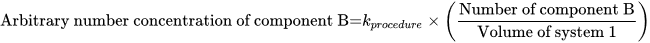Note: kprocedure is a constant defined by the measurement procedure (there is an approximately proportionality to a kind-of-quantity from the International System of Quantities)

### Result type

Ordinal scale
• Value set is ranked accoding to magnitude
• The value set and the limit of the value set are defined by the laboratory
• The value set can be either numerical values or words.Example 1Value set={none < some < high < very high}Example 2Value set={low < normal< high }Example 3

Value set={0 < 1 < 2}

Example 4

Value set={negative < positive}

Example 5

Value set={+ < ++ < +++}

• Algebraic operations are not allowed (Ref. 2, 1.26)
• Possible comparison of values (a<b, a=b or a>b)
Rational scale
• Value sets are positive numerical values
• Numerical values from 0-∞
• Algebraic operations are allowed
• Possible comparison of values (a=nb; a≠nb)

### Unit

International unit (IU) or procedure defined unit (p.d.u.) (non-SI units)

### Special rule(s) in the NPU syntax

“Procedure” is stated in the specification to the kind-of-property in the NPU definition.

“Procedure” indicates that the measurement procedure must be stated somewhere else than the NPU code and there may be difficulties in comparing values for the same NPU code.

If the ratio scale is attributed to the NPU definition, the unit can be non-SI unit.

If the unit is an International Unit (IU), an international certified reference material (e.g. WHO reference material) is specified in the specification to the kind-of-property.

If there exist no international certified reference material, the laboratory can specify the unit at the placeholder, procedure defined unit.

If ordinal scale is attributed, no unit is stated.

### Reference

1. Ferard G, Dybkaer R, Fuentes-Arderiu X. Compendium of Terminology and Nomenclature of Properties in Clinical Laboratory Sciences : Recommendations 2016. 1 ed: Royal Society of Chemistry; 2016. 182 p.
2. Mesures BIdPe. International Vocabulary of Metrology – Basic and General Concepts and Associated Terms VIM 3rd edition JCGM 200:2012 (JCGM 200:2008 with minor corrections). https://www.bipm.org: Bureau International des Poids et Mesures; 2008.

### Clinical Chemistry

Example 1 (ordinal)
Generic abbreviated form NPU29256 Marrow—Erythroid stem cell(EPO indep.); arb.num.c.(proc.) = ?
Full form with a result NPU29256 Marrow—Erythroid stem cell(EPO independent); arbitrary number concentration(procedure) = low
Abbreviated form with a result NPU29256 Marrow—Erythroid stem cell(EPO indep.); arb.num.c.(proc.) = low
Written expression The arbitrary number concentration of EPO independent Erythroid stem cell in Mr Smith’s Marrow is [low], compared with the arbitrary number concentration of EPO independent Erythroid stem cell in a reference system. The measurement is performed according to a procedure defined by the laboratory.
Notes
1. The reference system is defined by the laboratory.
2. “Low” is a value according to a value set defined by the laboratory.

### Clinical Microbiology

Example 2 (ratio)
Generic abbreviated form NPU28905 P—Hepatitis B virus(DNA); arb.num.c.(WHO 97/750; proc.) = ? × 10³ IU/L
Full form with a result NPU28905 Plasma—Hepatitis B virus(DNA); arbitrary number concentration(WHO 97/750; procedure) = 150 × 10³ IU/L
Abbreviated form with a result NPU28905 P—Hepatitis B virus(DNA); arb.num.c.(WHO 97/750; proc.) = 150 × 10³ IU/L
Written expression The arbitrary number concentration of Hepatitis B virus(DNA) in Mr Smith’s plasma is [1.5] × 10³ IU/L. The measurement is performed according to a procedure defined by the given laboratory. The measurement unit is defined by the Certified Reference Material, WHO 97/750.
Equation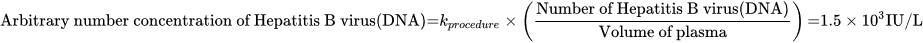Example 3 (ratio)
Generic abbreviated form NPU58268 P—Human immunodeficiency virus 1(RNA); arb.num.c.(proc.) = ? (p.d.u.)
Full form with a result NPU58268 Plasma—Human immunodeficiency virus 1(RNA); arbitrary number concentration(procedure) = 176 arbitrary unit/L
Abbreviated form with a result NPU58268 P—Human immunodeficiency virus 1(RNA); arb.num.c.(proc.) = 176 arbitrary unit/L
Written expression The arbitrary number concentration of Human immunodeficiency virus 1(RNA) in Mr Smith’s plasma is  arbitrary unit/L. The measurement is performed according to a procedure defined by the given laboratory.
Equation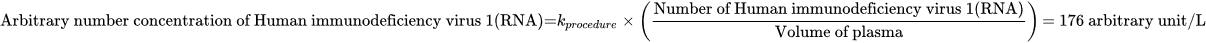Notes “Arbitrary unit” was chosen by a local laboratory as a proprietary measurement unit.and not an accepted international unit.

### Reproduction and Fertility

Example 4 (ratio)
Generic abbreviated form NPU18199 Sem—Spermatozoa; arb.num.c.(proc.) = ? (p.d.u.)
Full form with a result NPU18199 Semen—Spermatozoa; arbitrary number concentration(procedure) = 100 arbitrary unit/L
Abbreviated form with a result NPU18199 Sem—Spermatozoa; arb.num.c.(proc.) = 100 arbitrary unit/L
Written expression The arbitrary number concentration of Spermatozoa in Mr Smith’s semen is  arbitrary unit/L. The measurement is performed according to a procedure defined by the given laboratory.
Equation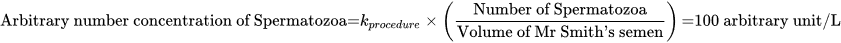Notes “Arbitrary unit” was chosen by a local laboratory as a proprietary measurement unit.and not an accepted international unit.
Example 5 (ratio)
Generic abbreviated form NPU54283 Sem—Spermatozoa(progressively motile); arb.num.c.(Direct swim-up; proc.) = ? (p.d.u.)
Full form with a result NPU54283 Semen—Spermatozoa(progressively motile); arb.num.c.(Direct swim-up; procedure) = 88 arbitrary unit/L
Abbreviated form with a result NPU54283 Sem—Spermatozoa(progressively motile); arb.num.c.(Direct swim-up; proc.) = 88 arbitrary unit/L
Written expression The arbitrary number concentration of Spermatozoa in Mr Smith’s semen is  arbitrary unit/L. The measurement is performed according to a procedure defined by the given laboratory.
Equation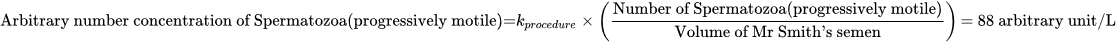Notes “Arbitrary unit” was chosen by a local laboratory as a proprietary measurement unit.and not an accepted international unit.

### Reference

NPU database, https://www.ifcc.org/ifcc-scientific-division/sd-committees/c-npu/npusearch/ and http://www.labterm.dk/default.aspx

• # Kind-of-property

• ## Kind-of-quantity

### Reference

• Dybkaer R. Concept system on ‘quantity’: formation and terminology. Accredit Qual Assur. 2013;18(3):253-60.
• Dybkaer R. ISO terminological analysis of the VIM3 concepts ‘quantity’ and ‘kind-of-quantity’. Metrologia. 2010;47(3):127-34.
Date Term Note
1996-01-01 Arbitrary number concentration Term established

QU50104